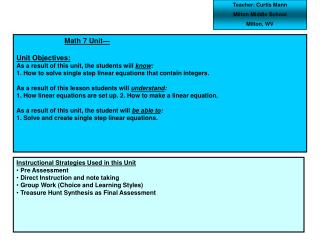Download PresentationMath 7 Unit— Unit Objectives: As a result of this unit, the students will know :

# Math 7 Unit— Unit Objectives: As a result of this unit, the students will know : - PowerPoint PPT PresentationDownload Presentation## Math 7 Unit— Unit Objectives: As a result of this unit, the students will know :

- - - - - - - - - - - - - - - - - - - - - - - - - - - E N D - - - - - - - - - - - - - - - - - - - - - - - - - - -
##### Presentation Transcript

1. Teacher: Curtis Mann Milton Middle School Milton, WV Math 7 Unit— Unit Objectives: As a result of this unit, the students will know: 1. How to solve single step linear equations that contain integers. As a result of this lesson students will understand: 1. How linear equations are set up. 2. How to make a linear equation. As a result of this unit, the student will be able to: 1. Solve and create single step linear equations. • Instructional Strategies Used in this Unit • Pre Assessment • Direct Instruction and note taking • Group Work (Choice and Learning Styles) • Treasure Hunt Synthesis as Final Assessment

2. Unit Outline

3. Acquisition Lesson Planning Form--Plan for the Concept, Topic, or Skill --- Not for the Day Essential Question: How much do you know about Linear Equations? Activating Strategy: Bell Ringer with Linear Equations not containing negative integers. While going over the Bell Ringer I remind them that in order to solve equations we must use the inverse operation to solve for the variable. Students will then complete Pre-Assessment. Teaching Strategies: Differentiated Instruction: None Summarizing Strategies:

4. Acquisition Lesson Planning Form--Plan for the Concept, Topic, or Skill --- Not for the Day Essential Question: How is solving equations with negative numbers alike/unlike solving equations with all positive numbers? Activating Strategy: We will begin by looking at notes previously taken on combining integers as well as Multiplying and Dividing integers. Teaching Strategies: Make students take out notes on Adding, Subtracting, Multiplying, and Dividing Integers for review, if need be give Individual Practice. Go over examples on Whiteboard, students are to copy into notes. Students will then complete some sort of practice (worksheet, bookwork, etc.) Differentiated Instruction: Ticket out the door - What is the one thing about solving these equations you are the most unclear on? Summarizing Strategies:

5. Acquisition Lesson Planning Form--Plan for the Concept, Topic, or Skill --- Not for the Day Essential Question: How can a Treasure Hunt help you learn to solve equations? Activating Strategy: Bell Ringer on one-step linear equations. Teaching Strategies: A thorough instruction on how the treasure hunt works. Students will then be paired according to there personality type, pairs will complete the treasure hunt. Differentiated Instruction: Ticket out the door – Which of the questions on the treasure hunt was the hardest? Summarizing Strategies:

6. Acquisition Lesson Planning Form--Plan for the Concept, Topic, or Skill --- Not for the Day Essential Question: How does the treasure hunt work? Activating Strategy: Go over the treasure hunt in order to show students how it can be made. Teaching Strategies: Students will be placed in groups according to their Learning Style. Students will then design a treasure hunt of their own, they have to create the problems themselves and place them in a treasure hunt. (See attached Rubric) This will serve as their final assessment for the Unit. Differentiated Instruction: Q & A Summary – Any questions about the creation of the treasure hunts that students might have. Summarizing Strategies:

7. Unit Title: One Step Linear Equations Containing Integers • CSOs ADDDRESED: • M.O.7.1.1 • M.O.7.1.3 • M.O.7.2.5 TEACHER NOTES Concepts: Students will have a solid foundation on adding, subtracting, Multiplying, and Dividing integers. Students will also get a good start on solving linear equations while gaining some problem solving skills. Generalizations: It is my experience tutoring at the college level that these concepts are 2 of the main tasks students tend to struggle with. If we can give them a solid foundation at this level it will make life easier not only for them but also the teachers they will have in the future.